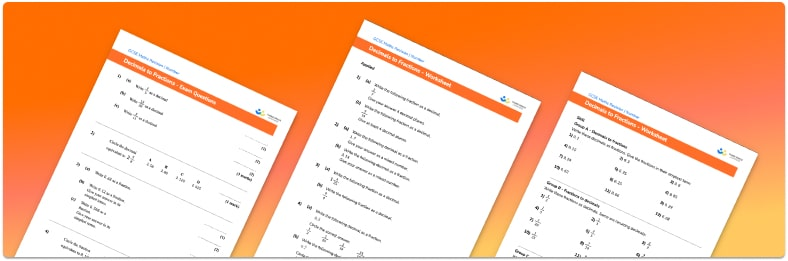# Converting Decimals To Fractions Worksheet• Section 1 of the converting decimals to fractions worksheet contains 36 skills-based converting decimals to fractions questions, in 3 groups to support differentiation
• Section 2 contains 4 applied converting decimals to fractions questions with a mix of word problems and deeper problem solving questions
• Section 3 contains 4 foundation and higher level GCSE exam style converting decimals to fractions questions
• Answer keys and a mark scheme for all converting decimals to fractions questions are provided
• Questions follow variation theory with plenty of opportunities for students to work independently at their own level
• All questions and math worksheets are created by fully qualified, expert secondary maths teachers
• Suitable for GCSE maths revision for AQA, OCR and Edexcel exam boards

• This field is for validation purposes and should be left unchanged.

You can unsubscribe at any time (each email we send will contain an easy way to unsubscribe). To find out more about how we use your data, see our privacy policy.

### Converting decimals to fractions at a glance

Fractions and decimals are different formats for representing a part of a whole. In order to convert decimal numbers to fractions we need to write the numbers after the decimal point as the numerator and then use the place value of the decimal to decide what the denominator will be. For example, if there is one decimal place (tenths), the denominator will be 10, if there are two decimal places (hundredths) the denominator will be 100 and so on. We are often asked to write a fraction in its simplest form so once we have a fraction we need to ensure it is simplified as much as possible.

To convert fractions to decimals we can either find an equivalent fraction with a denominator of 100 (then the numerator will represent the hundredths of the decimal), or we can divide the numerator by the denominator.

Students can then continue their understanding of converting decimals to fractions with additional number worksheets, for example aor aFor more teaching and learning support on Number our GCSE maths lessons provide step by step support for all GCSE maths concepts.

## Do you have KS4 students who need more focused attention to succeed at GCSE?There will be students in your class who require individual attention to help them succeed in their maths GCSEs. In a class of 30, it’s not always easy to provide.

Help your students feel confident with exam-style questions and the strategies they’ll need to answer them correctly with our dedicated GCSE maths revision programme.

Lessons are selected to provide support where each student needs it most, and specially-trained GCSE maths tutors adapt the pitch and pace of each lesson. This ensures a personalised revision programme that raises grades and boosts confidence.

Find out more